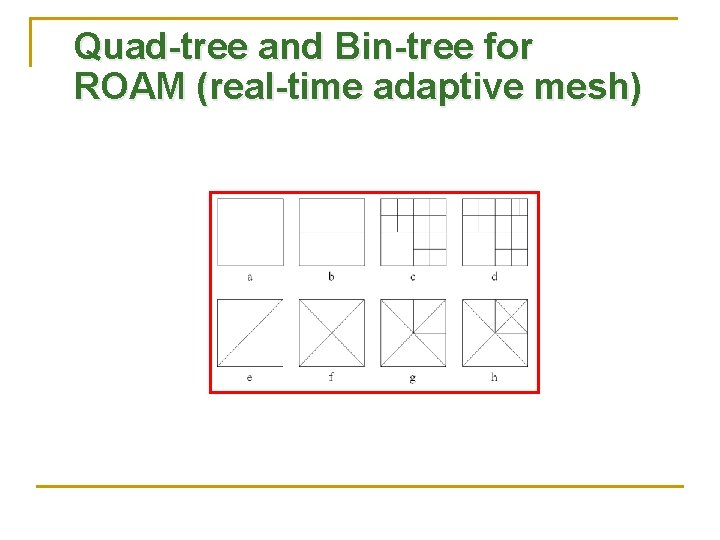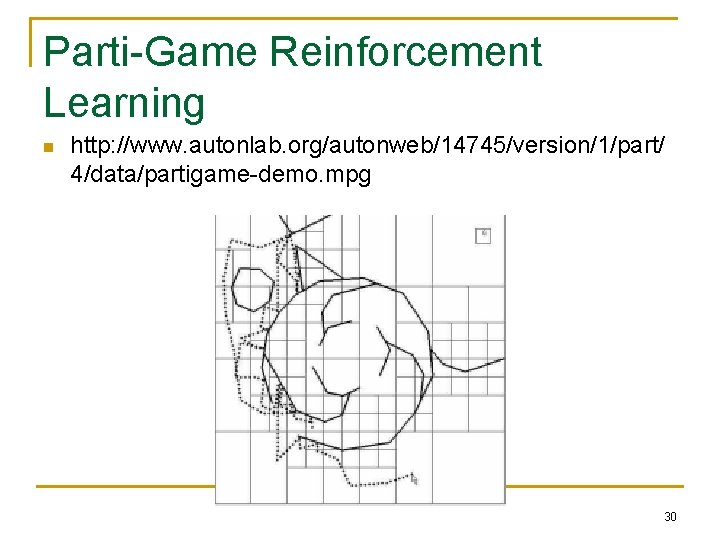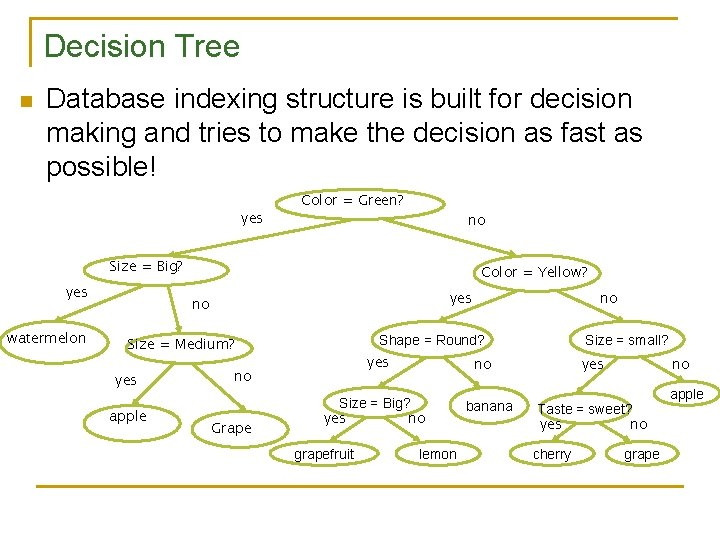# KD Trees Based on materials by Dennis Frey

• Slides: 28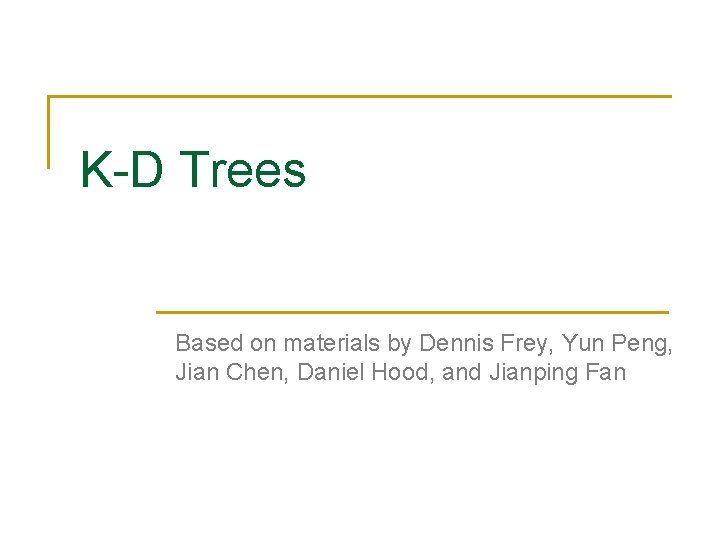K-D Trees Based on materials by Dennis Frey, Yun Peng, Jian Chen, Daniel Hood, and Jianping Fan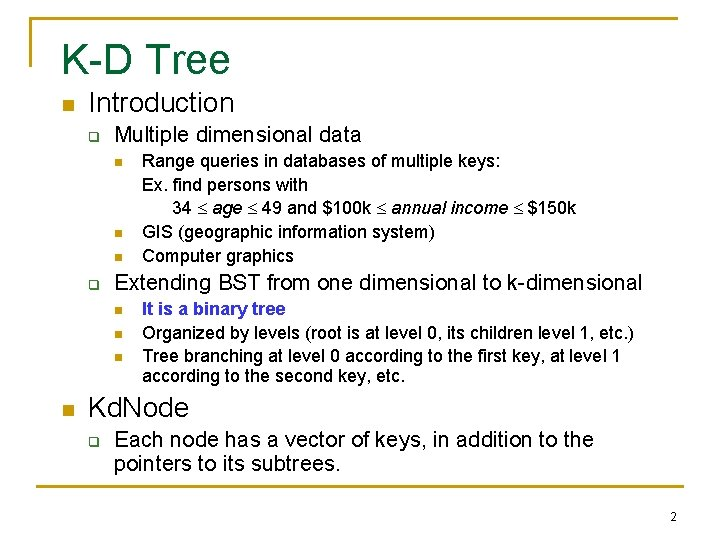K-D Tree n Introduction q Multiple dimensional data n n n q Extending BST from one dimensional to k-dimensional n n Range queries in databases of multiple keys: Ex. find persons with 34 age 49 and \$100 k annual income \$150 k GIS (geographic information system) Computer graphics It is a binary tree Organized by levels (root is at level 0, its children level 1, etc. ) Tree branching at level 0 according to the first key, at level 1 according to the second key, etc. Kd. Node q Each node has a vector of keys, in addition to the pointers to its subtrees. 2K-D Tree n A 2 -D tree example 3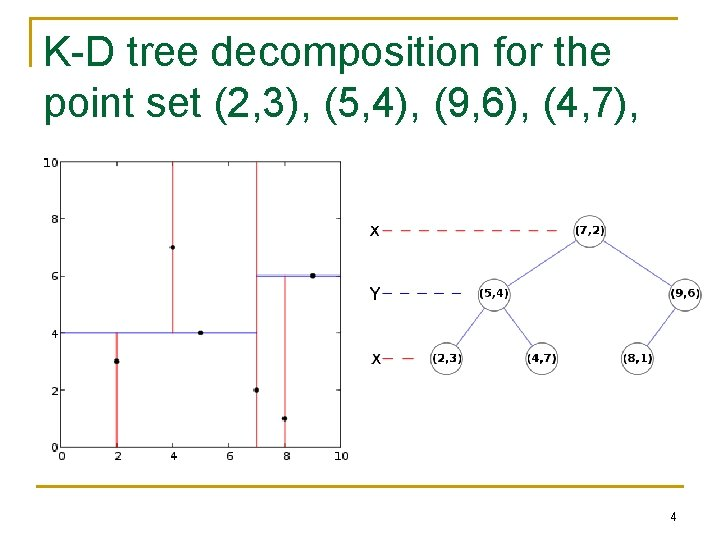K-D tree decomposition for the point set (2, 3), (5, 4), (9, 6), (4, 7), (8, 1), (7, 2). 4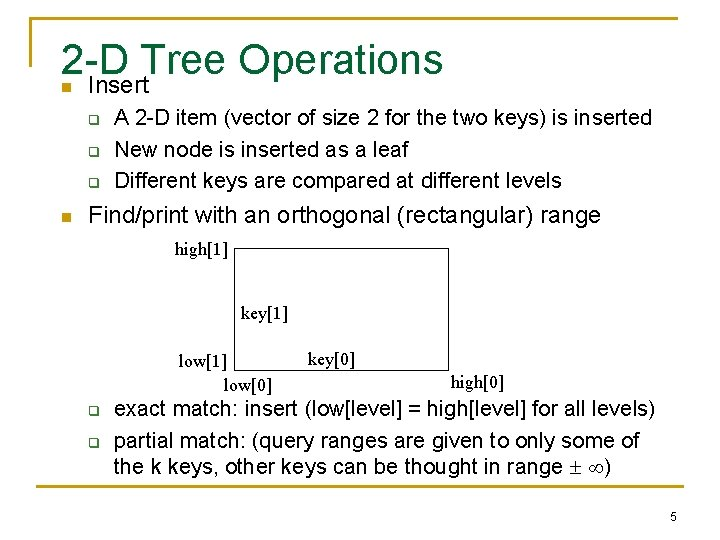2 -D Tree Operations Insert n q q q n A 2 -D item (vector of size 2 for the two keys) is inserted New node is inserted as a leaf Different keys are compared at different levels Find/print with an orthogonal (rectangular) range high key low q q key high exact match: insert (low[level] = high[level] for all levels) partial match: (query ranges are given to only some of the k keys, other keys can be thought in range ) 5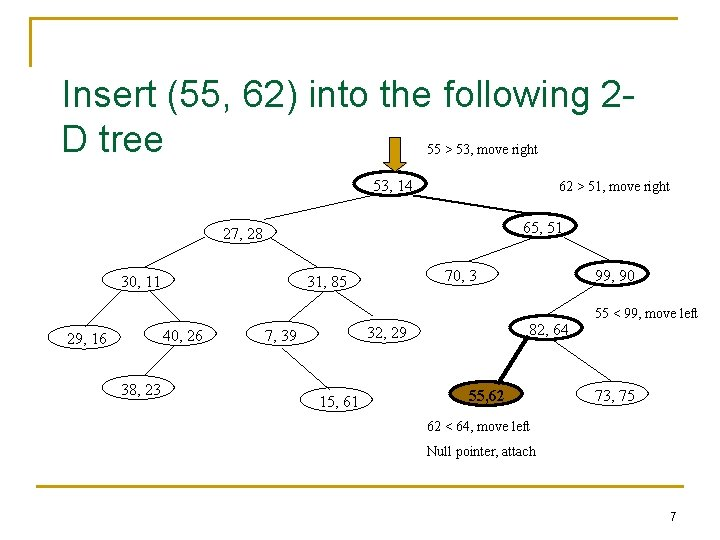Insert (55, 62) into the following 2 D tree 55 > 53, move right 53, 14 62 > 51, move right 65, 51 27, 28 30, 11 40, 26 29, 16 38, 23 70, 3 31, 85 82, 64 32, 29 7, 39 15, 61 99, 90 55, 62 55 < 99, move left 73, 75 62 < 64, move left Null pointer, attach 7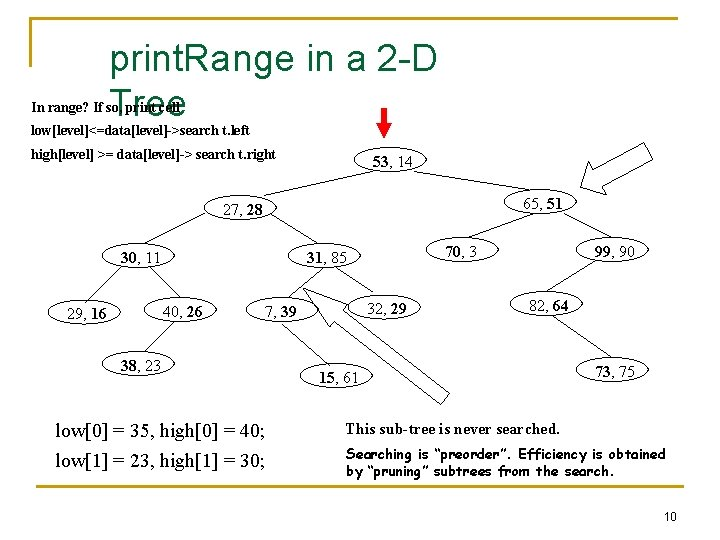print. Range in a 2 -D Tree In range? If so, print cell low[level]<=data[level]->search t. left high[level] >= data[level]-> search t. right 53, 14 65, 51 27, 28 30, 11 40, 26 29, 16 70, 3 31, 85 32, 29 7, 39 38, 23 low = 35, high = 40; low = 23, high = 30; 99, 90 82, 64 15, 61 73, 75 This sub-tree is never searched. Searching is “preorder”. Efficiency is obtained by “pruning” subtrees from the search. 10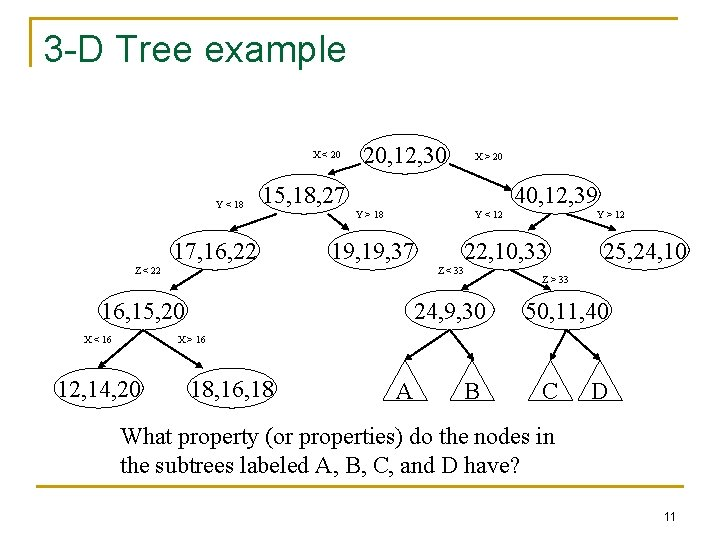3 -D Tree example X < 20 Y < 18 20, 12, 30 15, 18, 27 17, 16, 22 40, 12, 39 Y > 18 Y < 12 19, 37 Z < 22 Y > 12 22, 10, 33 Z < 33 16, 15, 20 X < 16 X > 20 24, 9, 30 25, 24, 10 Z > 33 50, 11, 40 X > 16 12, 14, 20 18, 16, 18 A B C D What property (or properties) do the nodes in the subtrees labeled A, B, C, and D have? 11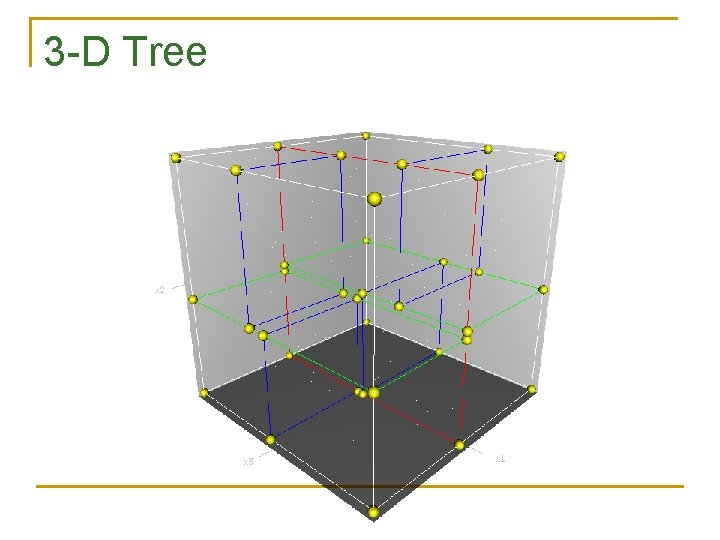3 -D TreeK-D Operations n n Modify the 2 -D insert code so that it works for K-D trees. Modify the 2 -D print. Range code so that it works for K-D trees. 13K-D Tree Performance n Insert q q n Average and balanced trees: O(lg N) Worst case: O(N) Print/search with a square range query q q Exact match: same as insert (low[level] = high[level] for all levels) Range query: for M matches n n Perfectly balanced tree: K-D trees: O(M + k. N (1 -1/k) ) 2 -D trees: O(M + N) Partial match in a random tree: O(M + N ) where = (-3 + 17) / 2 14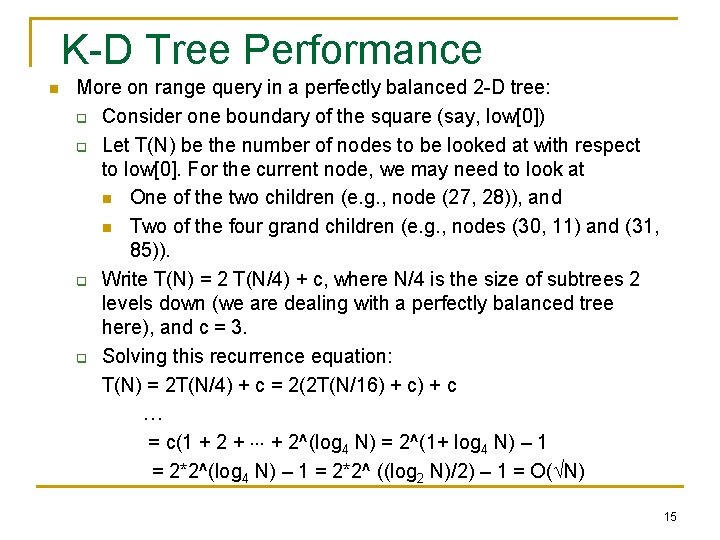K-D Tree Performance n More on range query in a perfectly balanced 2 -D tree: q Consider one boundary of the square (say, low) q Let T(N) be the number of nodes to be looked at with respect to low. For the current node, we may need to look at n One of the two children (e. g. , node (27, 28)), and n Two of the four grand children (e. g. , nodes (30, 11) and (31, 85)). q Write T(N) = 2 T(N/4) + c, where N/4 is the size of subtrees 2 levels down (we are dealing with a perfectly balanced tree here), and c = 3. q Solving this recurrence equation: T(N) = 2 T(N/4) + c = 2(2 T(N/16) + c … = c(1 + 2 + + 2^(log 4 N) = 2^(1+ log 4 N) – 1 = 2*2^(log 4 N) – 1 = 2*2^ ((log 2 N)/2) – 1 = O( N) 15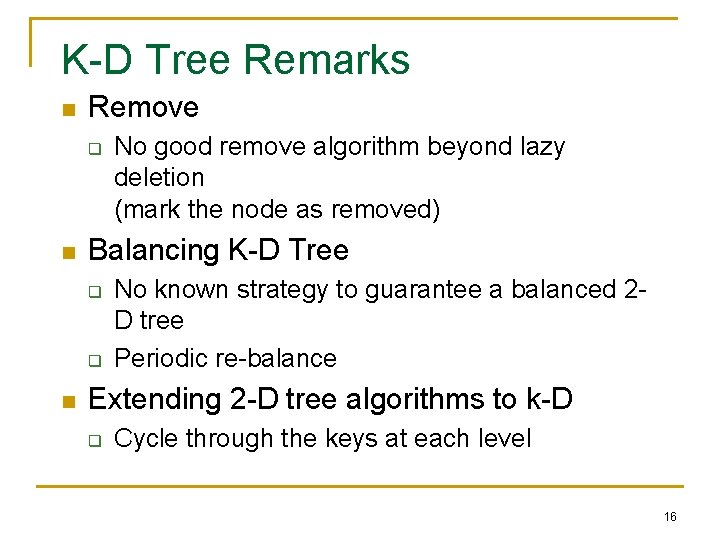K-D Tree Remarks n Remove q n Balancing K-D Tree q q n No good remove algorithm beyond lazy deletion (mark the node as removed) No known strategy to guarantee a balanced 2 D tree Periodic re-balance Extending 2 -D tree algorithms to k-D q Cycle through the keys at each level 16Insertion 20, 31 15, 15 36, 10 6, 6 31, 40 25, 16 Jaruloj Chongstitvatana k-d trees 17 40, 36Exact Search 20, 31 (40, 36) 15, 15 36, 10 6, 6 31, 40 25, 16 Jaruloj Chongstitvatana k-d trees 18 40, 36Range search 20, 31 15, 15 36, 10 6, 6 31, 40 25, 16 Jaruloj Chongstitvatana k-d trees 19 40, 36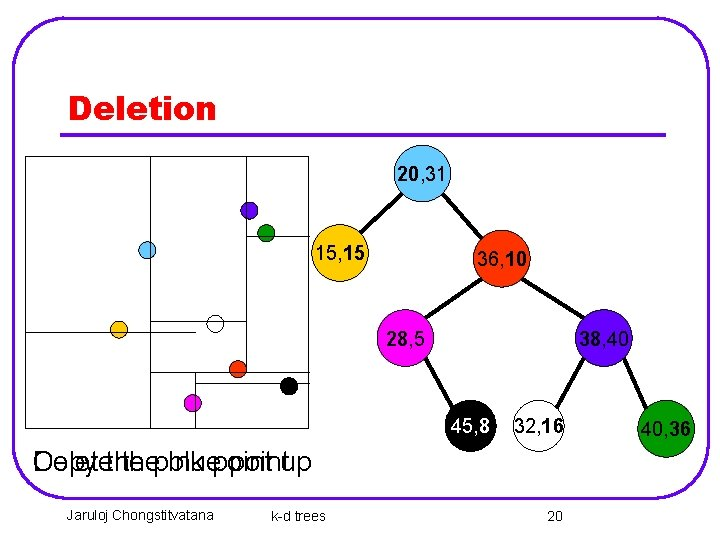Deletion 20, 31 15, 15 36, 10 28, 5 38, 40 45, 8 32, 16 Copy Deletethe thepink bluepointup Jaruloj Chongstitvatana k-d trees 20 40, 36Deletion 28, 5 15, 15 36, 10 38, 40 45, 8 32, 16 Delete the old pink point Jaruloj Chongstitvatana k-d trees 21 40, 36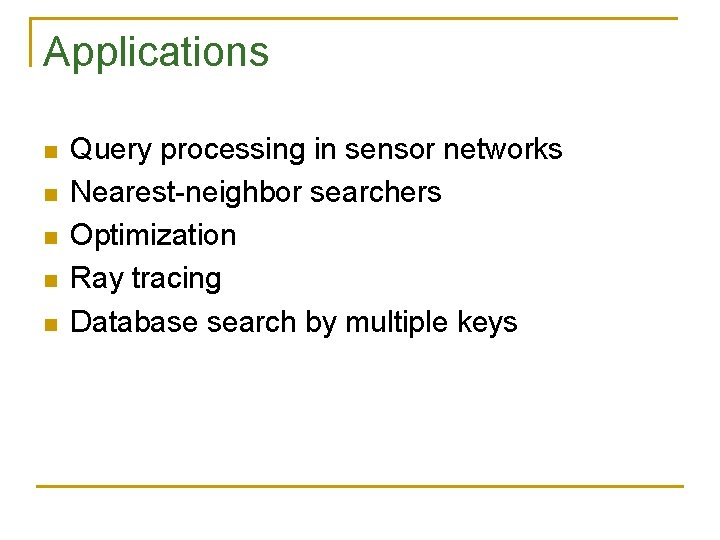Applications n n n Query processing in sensor networks Nearest-neighbor searchers Optimization Ray tracing Database search by multiple keysExamples of applications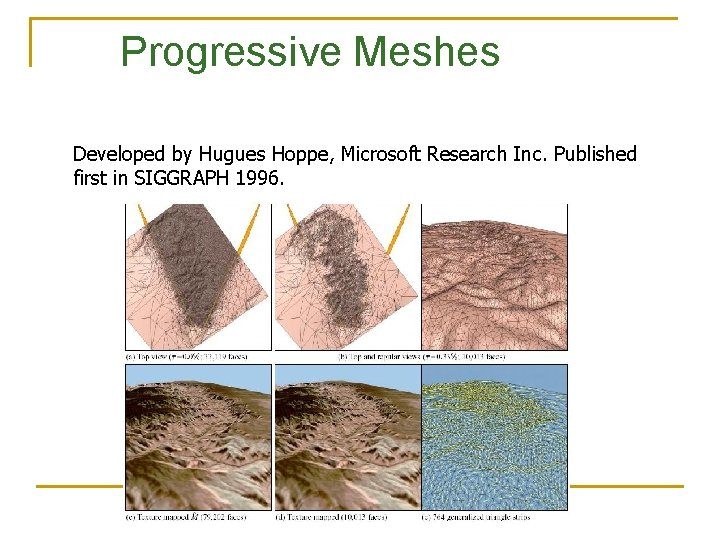Progressive Meshes Developed by Hugues Hoppe, Microsoft Research Inc. Published first in SIGGRAPH 1996.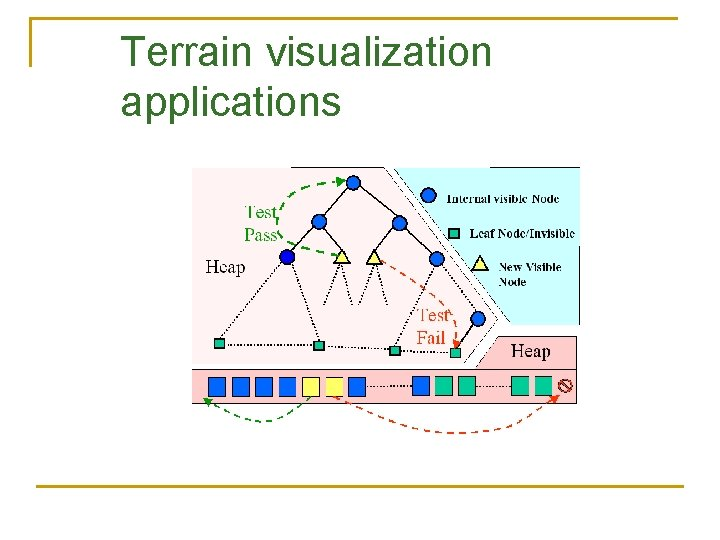Terrain visualization applicationsGeometric subdivision Problems with Geometric Subdivisions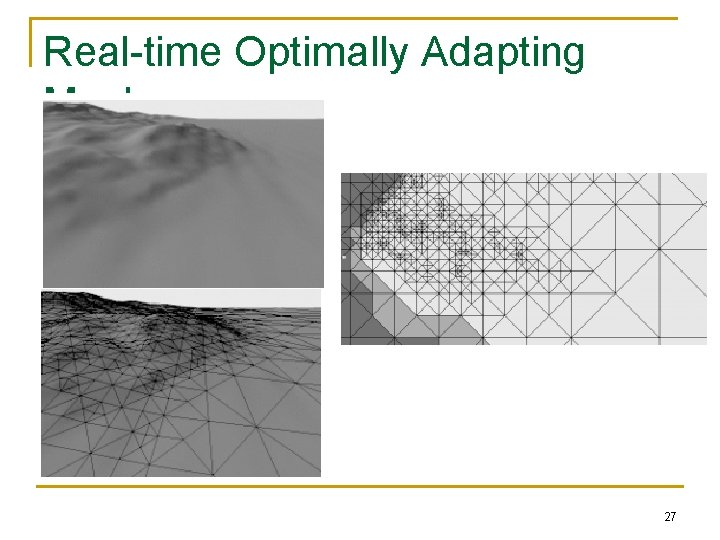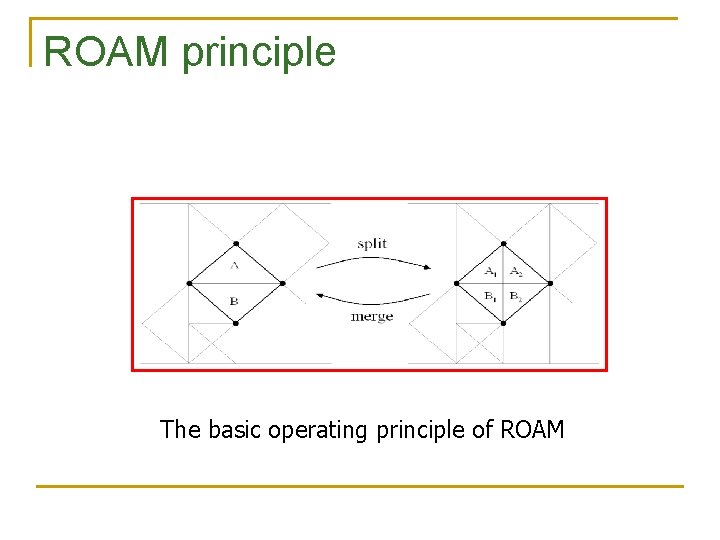ROAM principle The basic operating principle of ROAM